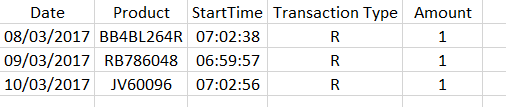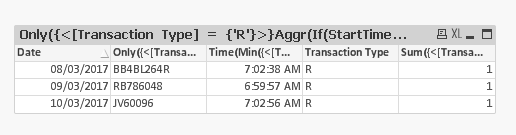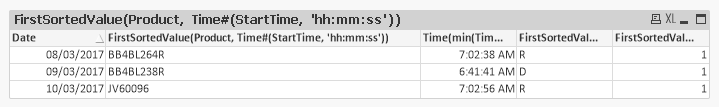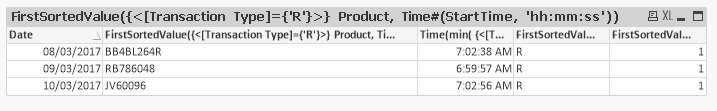# QlikView App Dev

Discussion Board for collaboration related to QlikView App Development.

cancel
Showing results for
Did you mean:Master II

## Firstsorted Value by Date and Time

Hi All,

I am trying to use an expression in a straight table to show the first transaction for each day.

I have the following TableAnd I want the following resultSo I need the first R transactions for each Day based on the time.

I hope you can help.

 Date Product StartTime Transaction Type Amount 08/03/2017 BB4BL264R 07:02:38 R 1 08/03/2017 MAAX94S 07:03:17 R 1 08/03/2017 RB783351 07:03:27 D 1 09/03/2017 BB4BL238R 06:41:41 D 1 09/03/2017 RB786048 06:59:57 R 1 09/03/2017 RB786055 07:00:14 R 1 10/03/2017 JV60096 07:02:56 R 1 10/03/2017 JV60096 07:02:59 R 1 10/03/2017 MP002676 07:03:17 R 1 Date Product StartTime Transaction Type Amount 08/03/2017 BB4BL264R 07:02:38 R 1 09/03/2017 RB786048 06:59:57 R 1 10/03/2017 JV60096 07:02:56 R 1
1 Solution

Accepted SolutionsMVP

Another option

Dimension

Date

Transaction Type

Expressions

Only({<[Transaction Type] = {'R'}>}Aggr(If(StartTime = Min(TOTAL <Date> {<[Transaction Type] = {'R'}>} StartTime), Only({<[Transaction Type] = {'R'}>}Product)), Product, Date, StartTime))

Time(Min({<[Transaction Type] = {'R'}>}Time#(StartTime,'hh:mm:ss')))

Sum({<[Transaction Type] = {'R'}>}Aggr(If(StartTime = Min(TOTAL <Date> {<[Transaction Type] = {'R'}>} StartTime), Sum({<[Transaction Type] = {'R'}>}Amount)), Product, Date, StartTime))

Used tresesco‌'s sample file to create a new chart (thanks)Best,

Sunny

19 RepliesMVPPFAMaster II
Author

That does not return the correct Data?MVP

Ohh, you wanted first transaction. Try removing '-' like:

FirstSortedValue(Product, Time#(StartTime, 'hh:mm:ss'))

for time min() instead of max().Master

Hi Alan,

Maybe:

Date Transaction Type Product StartTime Amount
08/03/2017RBB4BL264R07:02:381
09/03/2017RRB78604806:59:571
10/03/2017RJV6009607:02:56

1

Dims: Date and Transaction Type

Expressions:

Product: FirstSortedValue({\$<[Transaction Type] = {'R'}>}Product,StartTime)

StartTime:Min({\$<[Transaction Type] = {'R'}>}StartTime)

Amount:FirstSortedValue({\$<[Transaction Type] = {'R'}>}Amount,StartTime)

Cheers

AndrewMaster II
Author

It's almost there, I need to define a Transaction Type of R, at the moment I am getting the first transaction, but I need the first R transactionMVP

May be like:

FirstSortedValue({<[Transaction Type]={'R'}>} Product, Time#(StartTime, 'hh:mm:ss'))        , similarly for all

Time(min({<[Transaction Type={'R'}>} Time#(StartTime,'hh:mm:ss')))MVP

script solution

Data:

Product,

time(StartTime) as StartTime,

[Transaction Type],

Amount

FROM Table;

left join(Data)

time(min(StartTime)) as StartTime

1 as MinTimeFlag

resident Data

group by Date;

you can now write the expression like below

=sum({<MinTimeFlag={1}>}Amount)MVP

script solution

Data:

Product,

time(StartTime) as StartTime,

[Transaction Type],

Amount

FROM Table;

left join(Data)

time(min(StartTime)) as StartTime

1 as MinTimeFlag

resident Data

where [Transaction Type]='R'

group by Date;

you can now write the expression like below

=sum({<MinTimeFlag={1}>}Amount)Master II
Author

Hi,

I have tried each of the example and still can't get it.

Can I use in conjunction with Aggr, so I only have 1 expression and have Date, Product and StartTime as dimensions